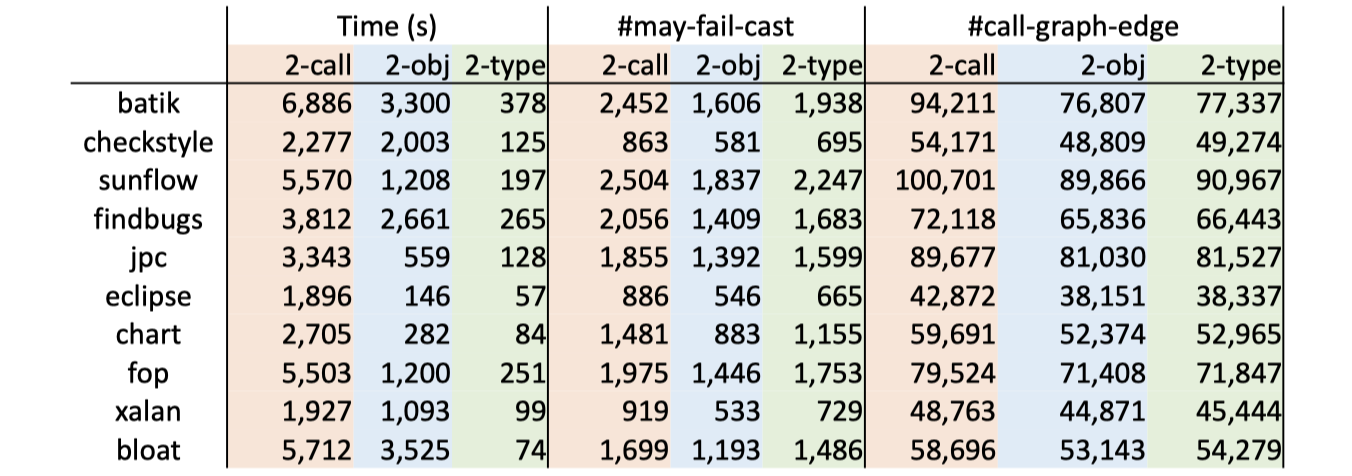## C.S.指针分析算法

$$\text{CSPointer} = (C \times V) \cup (C \times O \times F)$$

New i: x = new T() $\frac{}{c:o_{i} \in pt(c:x)}$ N/A
Assign x = y $\frac{c’:o_{i} \in pt(c:y)}{c’:o_{i} \in pt(c:x)}$ $c:x \leftarrow c:y$
Store x.f = y $\frac{c’:o_{i} \in pt(c:x),\ c’’:o_{j} \in pt(c:y)}{c’’:o_{j} \in pt(c’:o_{i}.f)}$ $c’:o_{i}.f \leftarrow c:y$
Load y = x.f $\frac{c’:o_{i} \in pt(c:x),\ c’’:o_{j} \in pt(c’:o_{i}.f)}{c’’:o_{j} \in pt(c:y)}$ $c:y \leftarrow c’:o_{i}.f$
Call l: r = x.k(a1, ..., an)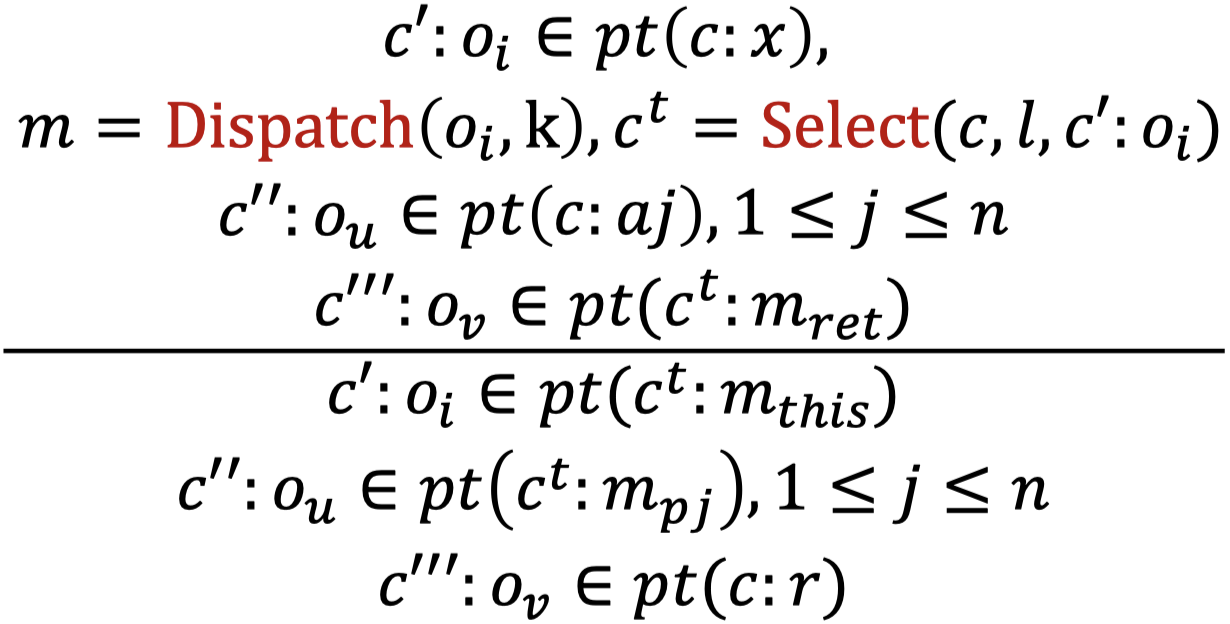$c:a1 \rightarrow c^{t}:m_{p1} \\ … \\ c:an \rightarrow c^{t}:m_{pn} \\ c:r \leftarrow c^{t}:m_{ret}$

OK，前菜结束，上算法：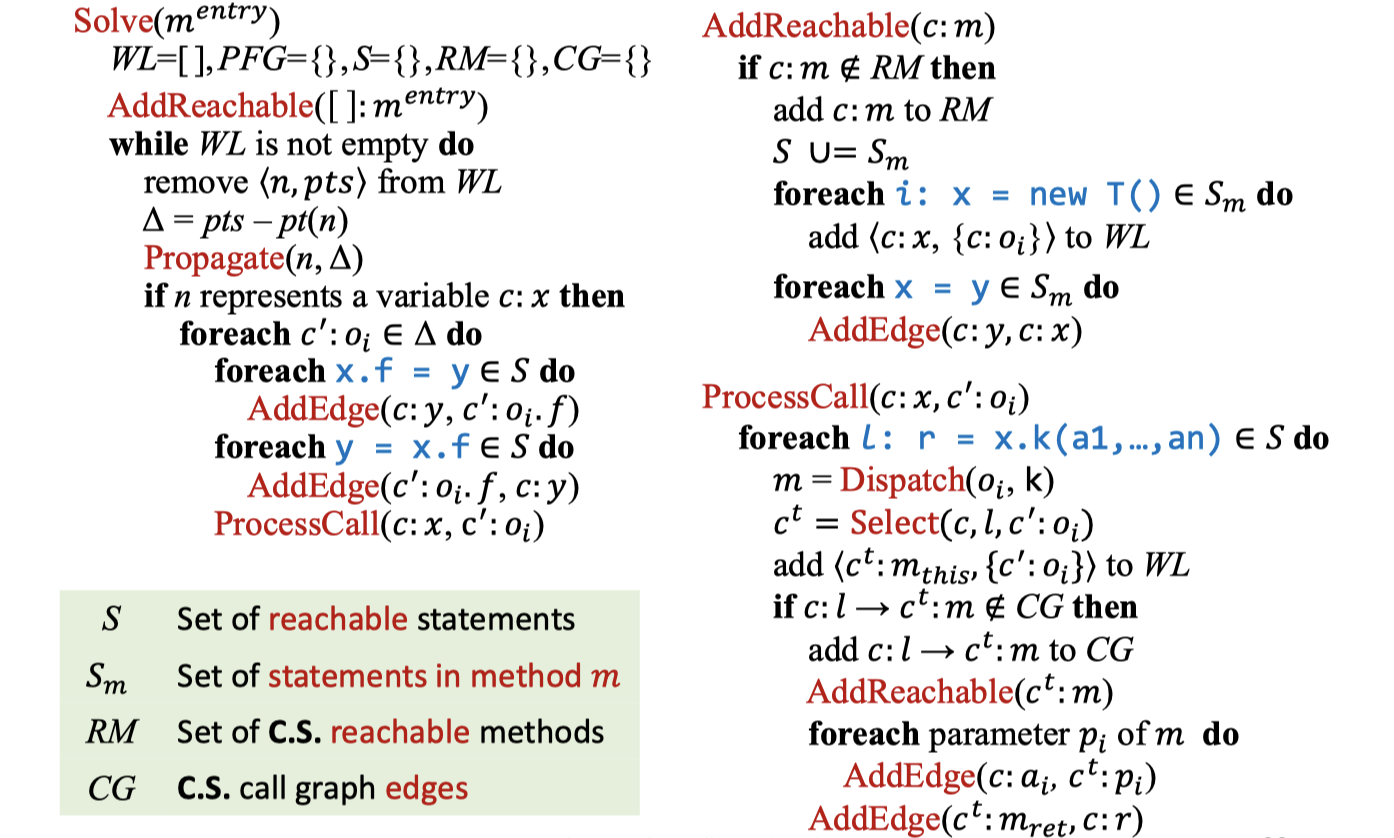C.I.的算法对比来看，C.S.的算法只是多了上下文限定（类似$c:$这样的部分）和$Select$函数。两个底层函数$AddEdge$和$Propagate$则在两个算法中完全相同——事实上，它俩从过程内C.I.指针分析开始就没变过。

## 不同类型的C.S.处理方式

$Select$函数的三个参数并非每次都是必需的。具体需要传入什么参数，要看C.S.处理方法是什么。我们还可以把C.I.指针分析纳入到C.S.中来，前者只是后者的一种特殊情况，相应的$Select$每次返回空（无上下文）即可。

OK，下面我们就来研究一下不同的C.S.处理方法。

### Call-Site Sensitivity

Call-site sensitivity由Olin Shivers于1991年在他的博士论文Control-Flow Analysis of Higher-Order Languages中提出。其思想是，每个上下文都是一个call sites列表（调用链），在方法调用时，将call site加入到caller context中，作为callee context，实质上就是调用栈的抽象： $$Select(c, l, \underline{\ \ }) = \lbrack l’,\ …\ , l’’, l \rbrack\ where\ c = \lbrack l’,\ …\ , l’’ \rbrack$$ 因为上下文形如一个字符串，因此call-site sensitivity也叫做call-string sensitivity，或者$k$-CFA。

$k$-CFA全称是k-Limiting Context Abstraction。顾名思义，就是要限制上下文的长度。Call-site sensitivity的特性导致这样产生的上下文可能会非常长，比如递归的情况。一方面，我们要确保指针分析能够终止；另一方面，我们不希望上下文过长，也没必要过长。

01 class c {
02     static void main() {
03         C c = new C();
04         c.m();
05     }
06
07     Number id(Number n) {
08         return n;
09     }
10     void m() {
11         Number n1, n2, x, y;
12         n1 = new One();
13         n2 = new Two();
14         x = this.id(n1);
15         y = this.id(n2);
16         x.get();
17     }
18 }


WL: []
PFG:
CG: {}
RM: { []:C.main() }


PFG:
[]:c{o_3}  :C.m_this{o_3}
:n1{o_12} -> :n{o_12} -> :x{o_12}
:n2{o_12} -> :n{o_13} -> :y{o_13}

CG: {
[]:4 -> :C.m(),  :14 -> :C.id(Number),
:15 -> :C.id(Number), :16 -> :One.get()
}


### Object Sensitivity

OK，接下来是第二种C.S.处理方式：对象敏感性。这种处理方式在2002年的一篇论文Parameterized Object Sensitivity for Points-to Analysis for Java被提出，思路与前一种截然不同——用抽象对象（由对象allocation sites表示）列表来构成上下文，而非call sites构成。在方法调用时，将接收者对象和它的heap context作为callee context。相应的$Select$函数如下：

$$Select(\underline{\ \ }, \underline{\ \ }, c’:o_{i}) = \lbrack o_{j},\ …\ , o_{k}, o_{i} \rbrack\ where\ c’ = \lbrack o_{j},\ …\ , o_{k} \rbrack$$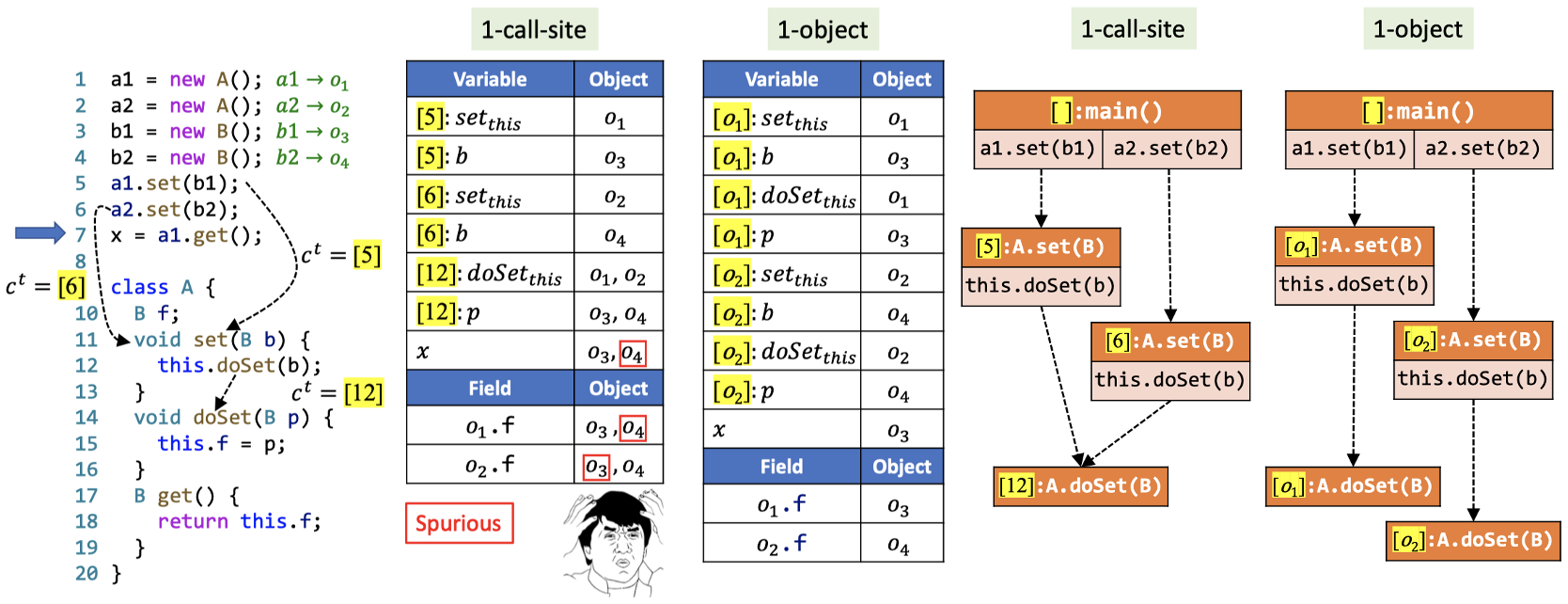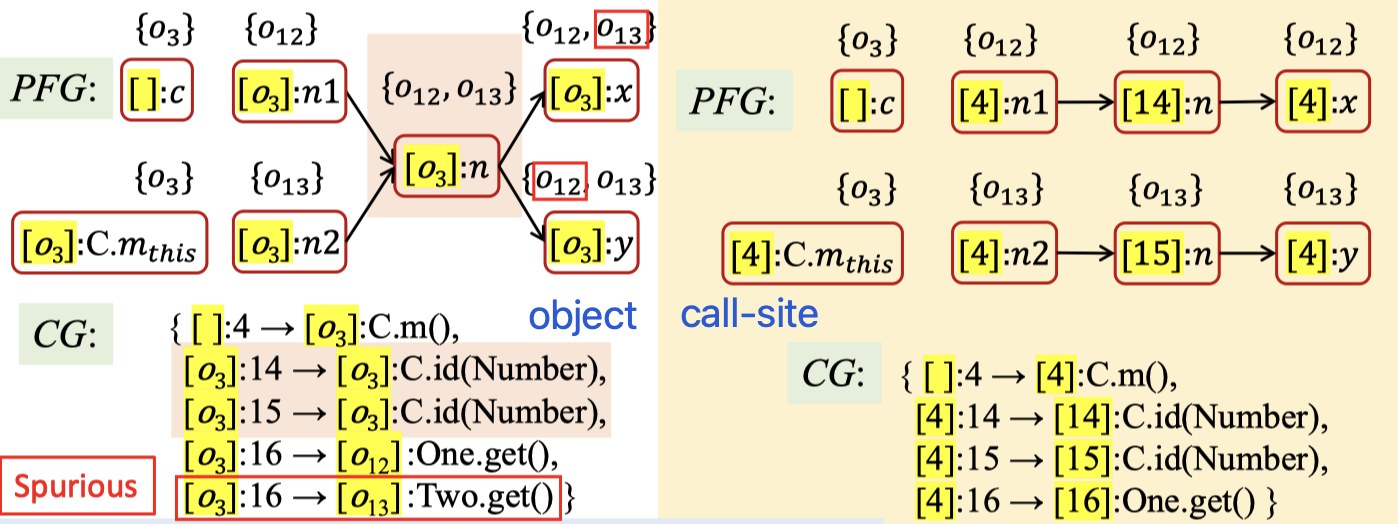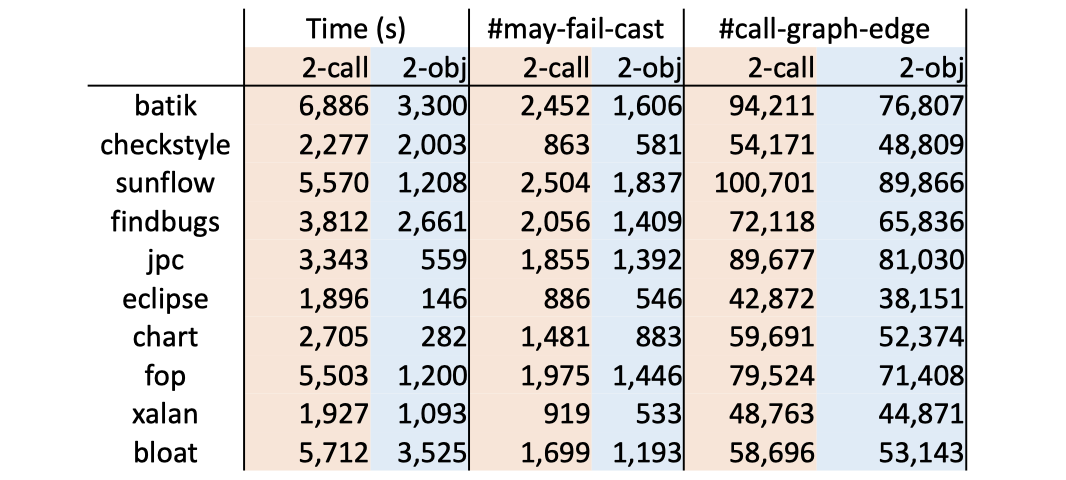### Type Sensitivity

$$Select(\underline{\ \ }, \underline{\ \ }, c’:o_{i}) = \lbrack t’,\ …\ , t’’, InType(o_{i}) \rbrack\ where\ c’ = \lbrack t’,\ …\ , t’’ \rbrack$$

1 class X {
2     void m() {
3         Y y = new Y();
4     }
5 }